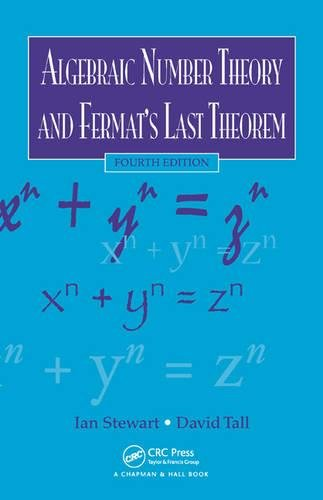# Algebraic Number Theory and Fermat’s Last Theorem, 4th Edition

• Length: 342 pages
• Edition: 4
• Publisher:
• Publication Date: 2015-10-13
• ISBN-10: 1498738397
• ISBN-13: 9781498738392
Description

Updated to reflect current research, Algebraic Number Theory and Fermat’s Last Theorem, Fourth Edition introduces fundamental ideas of algebraic numbers and explores one of the most intriguing stories in the history of mathematics―the quest for a proof of Fermat’s Last Theorem. The authors use this celebrated theorem to motivate a general study of the theory of algebraic numbers from a relatively concrete point of view. Students will see how Wiles’s proof of Fermat’s Last Theorem opened many new areas for future work.

New to the Fourth Edition

• Provides up-to-date information on unique prime factorization for real quadratic number fields, especially Harper’s proof that Z(√14) is Euclidean
• Presents an important new result: Mihăilescu’s proof of the Catalan conjecture of 1844
• Revises and expands one chapter into two, covering classical ideas about modular functions and highlighting the new ideas of Frey, Wiles, and others that led to the long-sought proof of Fermat’s Last Theorem
• Improves and updates the index, figures, bibliography, further reading list, and historical remarks

Written by preeminent mathematicians Ian Stewart and David Tall, this text continues to teach students how to extend properties of natural numbers to more general number structures, including algebraic number fields and their rings of algebraic integers. It also explains how basic notions from the theory of algebraic numbers can be used to solve problems in number theory.

Part I. Algebraic Methods
Chapter 1. Algebraic Background
Chapter 2. Algebraic Numbers
Chapter 3. Quadratic And Cyclotomic Fields
Chapter 4. Factorization Into Irreducibles
Chapter 5. Ideals

Part II. Geometric Methods
Chapter 6. Lattices
Chapter 7. Minkowski’S Theorem
Chapter 8. Geometric Representation Of Algebraic Numbers
Chapter 9. Class-Group And Class-Number

Part III. Number-Theoretic Applications
Chapter 10. Computational Methods
Chapter 11. Kummer’S Special Case Of Fermat’S Last Theorem
Chapter 12. The Path To The Final Breakthrough
Chapter 13. Elliptic Curves
Chapter 14. Elliptic Functions
Chapter 15. Wiles’S Strategy And Recent Developments

Part IV. Appendices